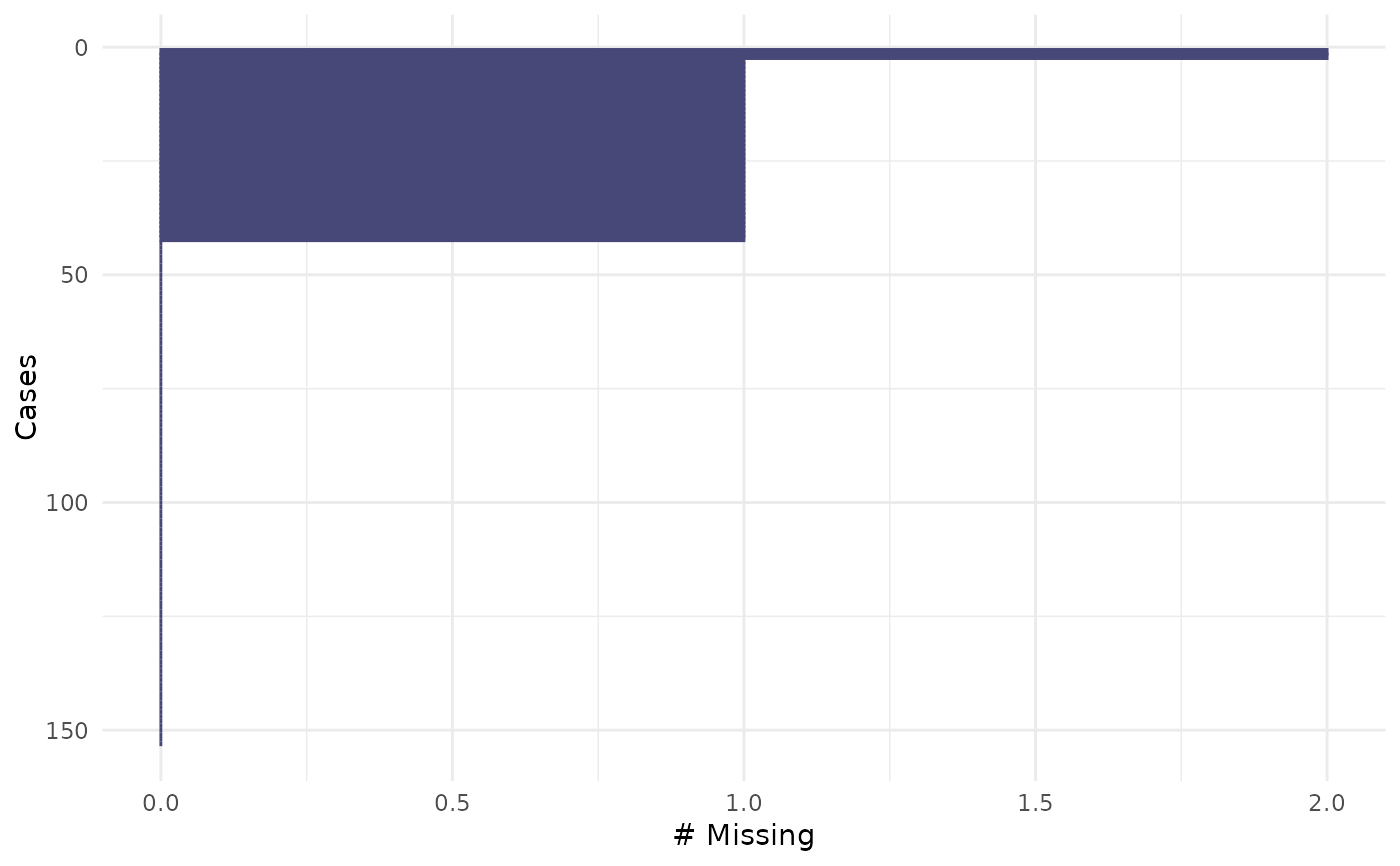This is a visual analogue to miss_case_summary. It draws a ggplot of the number of missings in each case (row). A default minimal theme is used, which can be customised as normal for ggplot.

## Usage

gg_miss_case(x, facet, order_cases = TRUE, show_pct = FALSE)

## Arguments

x

data.frame

facet

(optional) a single bare variable name, if you want to create a faceted plot.

order_cases

logical Order the rows by missingness (default is FALSE - no order).

show_pct

logical Show the percentage of cases

## Value

a ggplot object depicting the number of missings in a given case.

geom_miss_point() gg_miss_case_cumsum gg_miss_fct() gg_miss_span() gg_miss_var() gg_miss_var_cumsum() gg_miss_which()

## Examples


gg_miss_case(airquality)if (FALSE) {
library(ggplot2)
gg_miss_case(airquality) + labs(x = "Number of Cases")
gg_miss_case(airquality, show_pct = TRUE)
gg_miss_case(airquality, order_cases = FALSE)
gg_miss_case(airquality, facet = Month)
gg_miss_case(airquality, facet = Month, order_cases = FALSE)
gg_miss_case(airquality, facet = Month, show_pct = TRUE)
}